# imshow

## Syntax

``imshow(I)``
``imshow(I,[low high])``
``imshow(I,[])``
``imshow(RGB)``
``imshow(BW)``
``imshow(X,map)``
``imshow(filename)``
``imshow(___,Name,Value)``
``himage = imshow(___)``

## Description

example

````imshow(I)` displays the grayscale image `I` in a figure. `imshow` uses the default display range for the image data type and optimizes figure, axes, and image object properties for image display.```
````imshow(I,[low high])` displays the grayscale image `I`, specifying the display range as a two-element vector, `[low high]`. For more information, see the `DisplayRange` argument.```

example

````imshow(I,[])` displays the grayscale image `I`, scaling the display based on the range of pixel values in `I`. `imshow` uses `[min(I(:)) max(I(:))]` as the display range. `imshow` displays the minimum value in `I` as black and the maximum value as white. For more information, see the `DisplayRange` argument.```

example

````imshow(RGB)` displays the truecolor image `RGB` in a figure.```

example

````imshow(BW)` displays the binary image `BW` in a figure. For binary images, `imshow` displays pixels with the value `0` (zero) as black and `1` as white.```

example

````imshow(X,map)` displays the indexed image `X` with the colormap `map`.```

example

````imshow(filename)` displays the image stored in the graphics file specified by `filename`.```
````imshow(___,Name,Value)` displays an image, using name-value pairs to control aspects of the operation. ```
````himage = imshow(___)` returns the image object created by `imshow`.```

## Examples

collapse all

Display an RGB (truecolor), grayscale, binary, or indexed image using `imshow`.

Display an RGB Image

Read a sample RGB image, `peppers.png`, into the MATLAB workspace.

`rgbImage = imread("peppers.png");`

Display the RGB image using `imshow`.

`imshow(rgbImage)`Display a Grayscale Image

Convert the RGB image to a grayscale image by using the `rgb2gray` function.

`grayImage = rgb2gray(rgbImage);`

Display the grayscale image using `imshow`.

`imshow(grayImage)`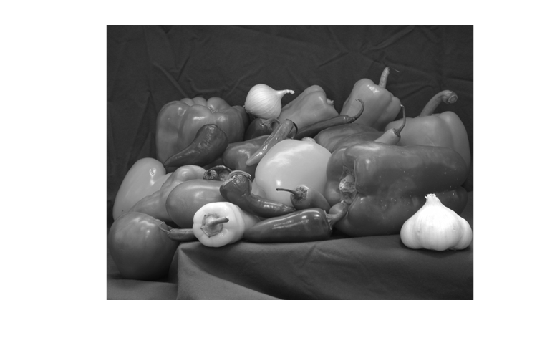Display a Binary Image

Convert the grayscale image to a binary image by using thresholding.

```meanVal = mean(grayImage,"all"); binaryImage = grayImage >= meanVal;```

Display the binary image using `imshow`.

`imshow(binaryImage)`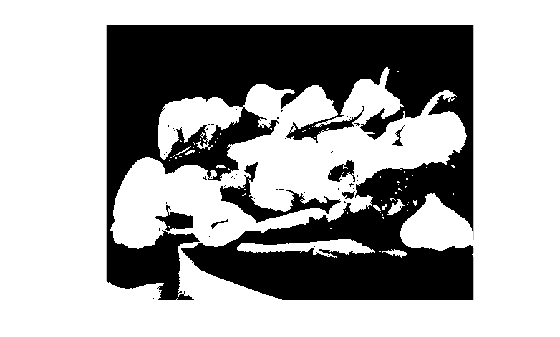Display an Indexed Image

Read a sample indexed image, `corn.tif`, into the MATLAB workspace.

`[corn_indexed,map] = imread('corn.tif');`

Display the indexed image using `imshow`.

`imshow(corn_indexed,map)`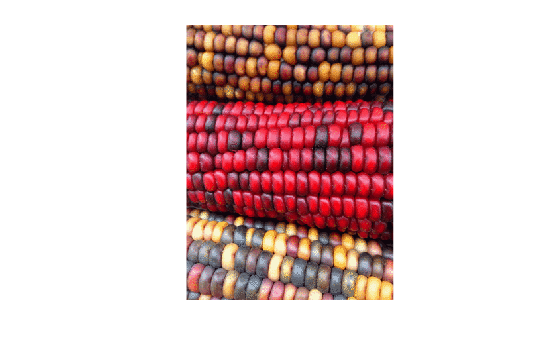Display an image stored in a file.

`imshow('peppers.png');`Load a sample grayscale volumetric image, `mri.mat`, into the variable `D` in the workspace. Remove the singleton dimension of the volume using the `squeeze` function.

```load("mri.mat"); vol = squeeze(D);```

Select a slice from the middle of the volume. Display the slice using the `copper` colormap and scaling the display range to the range of pixel values.

```sliceZ = vol(:,:,13); imshow(sliceZ,[],Colormap=copper)```Change the colormap for the image using the `colormap` function.

`colormap(hot)`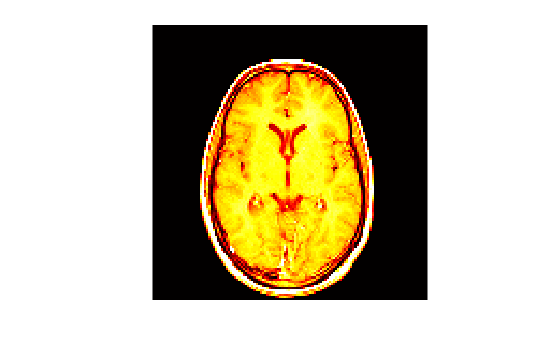Read a truecolor (RGB) image into the workspace. The data type of the image is `uint8`.

`RGB = imread('peppers.png');`

Extract the green channel of the image. The green channel is the second color plane.

```G = RGB(:,:,2); imshow(G)```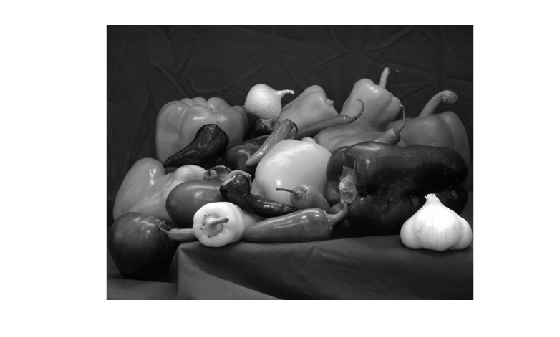Create a filter that detects horizontal edges in the image.

`filt = [-1 -1 -1;0 0 0;1 1 1];`

Filter the green channel of the image using the `filter2` function. The result is an image of data type `double`, with a minimum value of -422 and a maximum value of 656. Pixels with a large magnitude in the filtered image indicate strong edges.

`edgeG = filter2(filt,G);`

Display the filtered image using `imshow` with the default display range. For images of data type `double`, the default display range is [0, 1]. The image appears black and white because the filtered pixel values exceed the range [0, 1].

`imshow(edgeG)`Display the filtered image and scale the display range to the pixel values in the image. The image displays with the full range of grayscale values.

`imshow(edgeG,[])`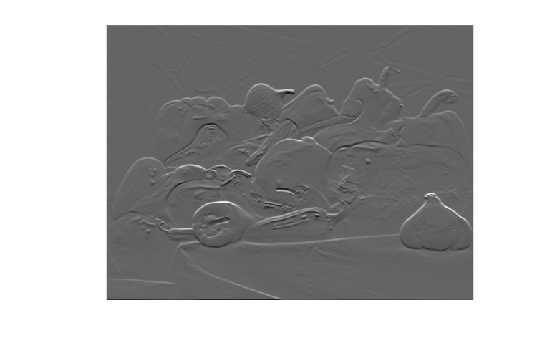Read the grayscale image from the `corn.tif` file into the workspace. The grayscale version of the image is the second image in the file.

`corn_gray = imread('corn.tif',2);`

Select a small portion of the image. Display the detail image at 100% magnification using `imshow`.

```corn_detail = corn_gray(1:100,1:100); imshow(corn_detail)```Display the image at 1000% magnification by using the `'InitialMagnification'` name-value pair argument. By default, `inshow` performs nearest neighbor interpolation of pixel values. The image has blocking artifacts.

`imshow(corn_detail,'InitialMagnification',1000)`Display the image at 1000% magnification, specifying the bilinear interpolation technique. The image appears smoother.

`imshow(corn_detail,'InitialMagnification',1000,'Interpolation',"bilinear")`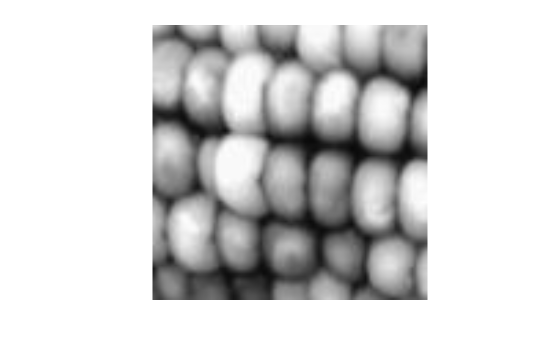## Input Arguments

collapse all

Input grayscale image, specified as a matrix. A grayscale image can be any numeric data type.

Data Types: `single` | `double` | `int8` | `int16` | `int32` | `int64` | `uint8` | `uint16` | `uint32` | `uint64` | `logical`

Input truecolor image, specified as an m-by-n-by-3 array.

If you specify a truecolor image of data type `single` or `double`, then values should be in the range [0, 1]. If pixel values are outside this range, then you can use the `rescale` function to scale pixel values to the range [0, 1]. The `'DisplayRange'` argument has no effect when the input image is truecolor.

Data Types: `single` | `double` | `uint8` | `uint16`

Input binary image, specified as a matrix.

Data Types: `logical`

Indexed image, specified as a 2-D matrix of positive integers. The values in `X` are indices into the colormap specified by `map`.

Data Types: `single` | `double` | `uint8` | `logical`

Colormap associated with indexed image `X`, specified as a c-by-3 matrix. Each row of `map` is a three-element RGB triplet that specifies the red, green, and blue components of a single color of the colormap. When `map` is of data type `single` or `double`, the values of the matrix are in the range [0, 1].

Data Types: `single` | `double` | `uint8`

File name, specified as a character vector. The image must be readable by the `imread` function. The `imshow` function displays the image, but does not store the image data in the MATLAB® workspace. If the file contains multiple images, then `imshow` displays the first image in the file.

Example: `'peppers.png'`

Data Types: `char`

Grayscale image display range, specified as a two-element vector. For more information, see the `'DisplayRange'` name-value pair argument.

Example: `[50 250]`

Data Types: `single` | `double` | `int8` | `int16` | `int32` | `int64` | `uint8` | `uint16` | `uint32` | `uint64`

### Name-Value Arguments

Specify optional pairs of arguments as `Name1=Value1,...,NameN=ValueN`, where `Name` is the argument name and `Value` is the corresponding value. Name-value arguments must appear after other arguments, but the order of the pairs does not matter.

Before R2021a, use commas to separate each name and value, and enclose `Name` in quotes.

Example: `imshow('board.tif','Border','tight')`

Figure window border space, specified as the comma-separated pair consisting of `'Border'` and either `'tight'` or `'loose'`. When set to `'loose'`, the figure window includes space around the image in the figure. When set to `'tight'`, the figure window does not include any space around the image in the figure.

If the image is very small or if the figure contains other objects besides an image and its axes, `imshow` might use a border regardless of how this parameter is set.

Data Types: `char`

Colormap of the axes, specified as the comma-separated pair consisting of `'Colormap'` and a c-by-3 matrix with values in the range [0, 1]. Each row of the matrix is a three-element RGB triplet that specifies the red, green, and blue components of a single color of the colormap. Use this argument to view grayscale images in false color. If you specify an empty colormap (`[]`), then the `imshow` function ignores this argument.

Example: ```cmap = copper; imshow('board.tif','Colormap',cmap)```

Data Types: `double`

Display range of a grayscale image, specified as a two-element vector of the form `[low high]`. The `imshow` function displays the value `low` (and any value less than `low`) as black, and it displays the value `high` (and any value greater than `high`) as white. Values between `low` and `high` are displayed as intermediate shades of gray, using the default number of gray levels.

If you specify an empty matrix (`[]`), then `imshow` uses a display range of `[min(I(:)) max(I(:))]`. In other words, the minimum value in `I` is black, and the maximum value is white.

If you do not specify a display range, then `imshow` selects a default display range based on the image data type.

• If `I` is an integer type, then `DisplayRange` defaults to the minimum and maximum representable values for that integer class. For example, the default display range for `uint16` arrays is [0, 65535].

• If `I` is data type `single` or `double`, then the default display range is [0, 1].

Note

Including the parameter name is optional, except when the image is specified by a file name. The syntax ```imshow(I,[low high])``` is equivalent to `imshow(I,'DisplayRange',[low high])`. If you call `imshow` with a file name, then you must specify the `'DisplayRange'` parameter.

Example: `'DisplayRange',[10 250]`

Data Types: `single` | `double` | `int8` | `int16` | `int32` | `int64` | `uint8` | `uint16` | `uint32` | `uint64`

Initial magnification of the image display, specified as the comma-separated pair consisting of `'InitialMagnification'` and a numeric scalar or `'fit'`. If set to `100`, then `imshow` displays the image at 100% magnification (one screen pixel for each image pixel). If set to `'fit'`, then `imshow` scales the entire image to fit in the window.

Initially, `imshow` attempts to display the entire image at the specified magnification. If the magnification value is so large that the image is too big to display on the screen, `imshow` displays the image at the largest magnification that fits on the screen.

If the image is displayed in a figure with its `'WindowStyle'` property set to `'docked'`, then `imshow` displays the image at the largest magnification that fits in the figure.

Note: If you specify the axes position, `imshow` ignores any initial magnification you might have specified and defaults to the `'fit'` behavior.

When you use `imshow` with the `'Reduce'` parameter, the initial magnification must be `'fit'`.

In MATLAB Online™, `'InitialMagnification'` is set to `'fit'` and cannot be changed.

Example: `'InitialMagnification',80`

Data Types: `single` | `double` | `int8` | `int16` | `int32` | `int64` | `uint8` | `uint16` | `uint32` | `uint64` | `char`

Interpolation method, specified as `'nearest'` or `'bilinear'`. MATLAB uses interpolation to display a scaled version of the image on your screen. The value you choose does not affect the image data. Choose an interpolation method based on your image content and the effect you want to achieve:

• `'nearest'` — Nearest neighbor interpolation. The value of a pixel located at (x, y) is the value of the pixel that is closest to (x, y) in the original image. This method is best when there are a small number of pixel values that represent distinct categories, or when you want to see individual pixels in a highly zoomed-in view.

• `'bilinear'` — Bilinear interpolation. The value of a pixel located at (x, y) is a weighted average of the surrounding pixels in the original image. To minimize display artifacts, the `imshow` function performs antialiasing when you shrink the image. This method is best in almost all other situations.

Parent axes of image object, specified as the comma-separated pair consisting of `'Parent'` and an `Axes` object or a `UIAxes` object. Use the `'Parent'` name-value argument to build a UI that gives you control of the `Figure` and `Axes` properties.

Indicator for subsampling image, specified as the comma-separated pair consisting of `'Reduce'` and either `true`, `false`, `1`, or `0`. This argument is valid only when you use it with the name of a TIFF file. Use the `Reduce` argument to display overviews of very large images.

Data Types: `logical`

X-axis limits of nondefault coordinate system, specified as the comma-separated pair consisting of `'XData'` and a two-element vector. This argument establishes a nondefault spatial coordinate system by specifying the image `XData`. The value can have more than two elements, but `imshow` uses only the first and last elements.

Example: `'XData',[100 200]`

Data Types: `single` | `double` | `int8` | `int16` | `int32` | `int64` | `uint8` | `uint16` | `uint32` | `uint64`

Y-axis limits of nondefault coordinate system, specified as the comma-separated pair consisting of `'YData'` and a two-element vector. The value can have more than two elements, but `imshow` uses only the first and last elements.

Example: `'YData',[100 200]`

Data Types: `single` | `double` | `int8` | `int16` | `int32` | `int64` | `uint8` | `uint16` | `uint32` | `uint64`

## Output Arguments

collapse all

Image created by the `imshow` function, specified as an image object.

## Tips

• To change the colormap after you create the image, use the `colormap` command.

• You can display multiple images with different colormaps in the same figure using `imshow` with the `tiledlayout` and `nexttile` functions.

• You can create an axes on top of the axes created by `imshow` by using the `hold on` command after calling `imshow`.

• The `imshow` function is not supported when you start MATLAB with the `-nojvm` option.

## Version History

Introduced before R2006a